# Functional Decomposition Functional Decomposition Functional Decomposition RothKarp Decomposition

• Slides: 9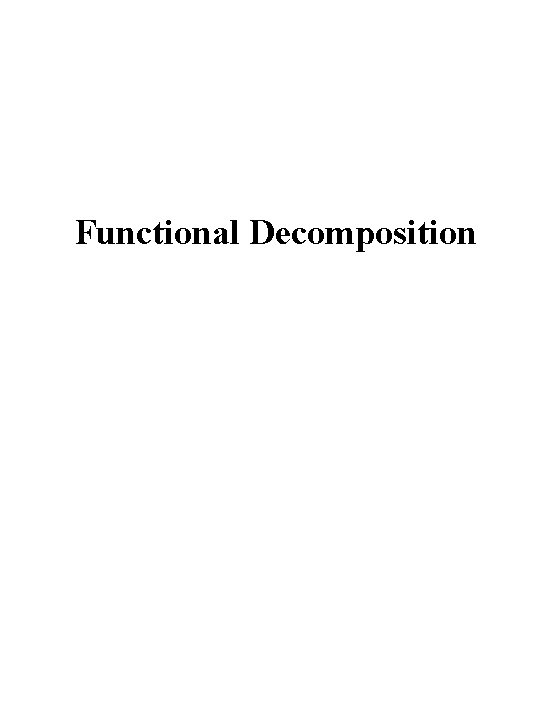Functional Decomposition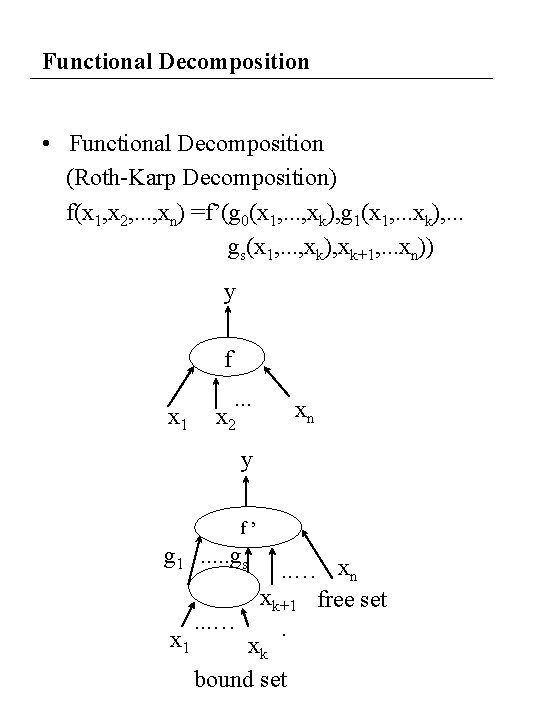Functional Decomposition • Functional Decomposition (Roth-Karp Decomposition) f(x 1, x 2, . . . , xn) =f’(g 0(x 1, . . . , xk), g 1(x 1, . . . xk), . . . gs(x 1, . . . , xk), xk+1, . . . xn)) y f x 1 . . . xn x 2 y f’ g 1. . . gs x 1 . . . … xn xk+1 free set. xk bound set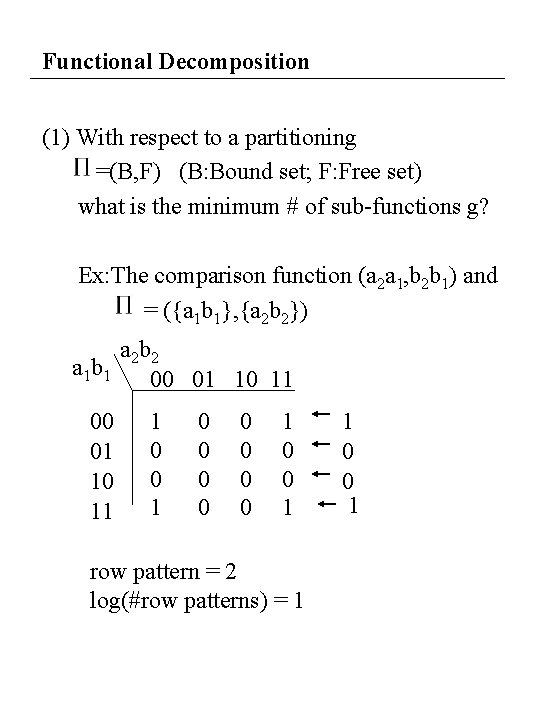Functional Decomposition (1) With respect to a partitioning =(B, F) (B: Bound set; F: Free set) what is the minimum # of sub-functions g? Ex: The comparison function (a 2 a 1, b 2 b 1) and = ({a 1 b 1}, {a 2 b 2}) a 2 b 2 a 1 b 1 00 01 10 11 1 0 0 0 0 0 1 row pattern = 2 log(#row patterns) = 1 1 0 0 1Functional Decomposition 1. x 1 x 2 for x 1, x 2 B if f (x 1, y) = f (x 2, y) for all y F x 1 and x 2 are in the same equivalence class 2. The number of row patterns is the number of equivalence class 3. The minimum number of g functions is log ( the number of equivalent classes)Example (2) How to synthesize g , f’ ? Ex: a 2 b 200 01 10 11 a 1 b 1 00 1 0 0 1 1 01 0 0 0 10 0 0 11 1 0 0 1 f’(g a 2 b 2) g 00 01 10 11 1 1 0 0 0 0 0 g(a 1 b 1) a 1 b 1 g 00 1 01 0 10 0 11 1 f’ g a 2 b 2 a 1 b 1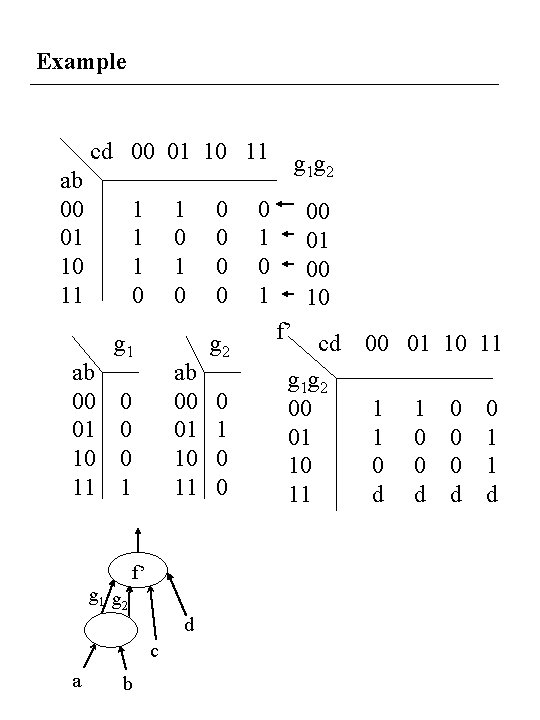Example cd 00 01 10 11 ab 00 01 10 11 1 0 g 1 ab 00 01 10 11 0 0 0 1 f’ g 1 g 2 d c a b 0 0 g 2 0 1 0 0 g 1 g 2 0 1 00 01 00 10 f’ cd g 1 g 2 00 01 10 11 1 1 0 d 1 0 0 d 0 1 1 d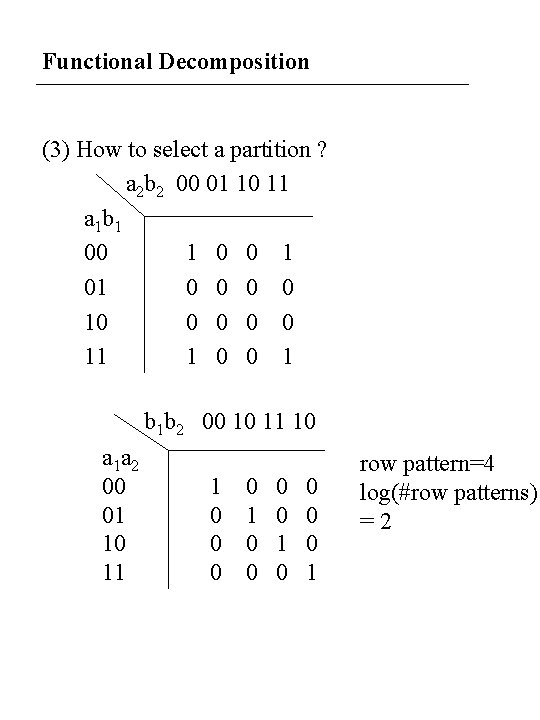Functional Decomposition (3) How to select a partition ? a 2 b 2 00 01 10 11 a 1 b 1 00 1 01 0 0 10 0 0 11 1 0 0 1 b 1 b 2 00 10 11 10 a 1 a 2 00 01 10 11 1 0 0 0 0 1 row pattern=4 log(#row patterns) =2Example • Compute the number of equivalence classes using BDD x 2 x 3 00 01 10 11 x 0 x 1 00 0 0 1 0 A 01 0 0 1 1 B 10 0 0 1 0 A 11 0 0 1 0 AExample(cont. ) x 0 0 1 B x 2 0 x 1 1 1 0 0 1 0 x 2 A 1 x 3 0 1 1. Order BDD such that the variables in the bound set is on the top of BDD 2. Draw a line between the bound set and the free set 3. The number of equivalence classes is the number of sub-trees rooted at the line